# JEE Advanced 2019 Chemistry Questions Paper 1

JEE Advanced 2019 Chemistry Paper 1 solutions are available here. All questions are solved by subject experts and in a detailed manner. Students can also download the solved paper in a PDF format for offline practice to improve their speed and accuracy. Practising JEE Advanced 2019 Chemistry question Paper 1 will help the aspirants to understand the overall exam pattern and prepare accordingly for upcoming exam.

### Paper 1 - Chemistry

1. Which of the following set represent correct formula for Malachite, Magnetite, Calamine & Cryolite?

1. a) CuCO3, Fe2O3, ZnO, Al2O3
2. b) CuCO3,Cu(OH), Fe3O4, ZnCO3, Na3 AlF6
3. c) CuCO3, Fe3O4, ZnCO3, Al2O3
4. d) CuCO3.Cu(OH), Fe2O3, ZnCO3, Na3AlF6

Solution:

1. Malachite → CuCO3.Cu(OH)2

Magnetite → Fe3O4

Calamine → ZnCO3

Cryollite → Na3AlF6

2.The correct order of acid strength of the following carboxylic acids is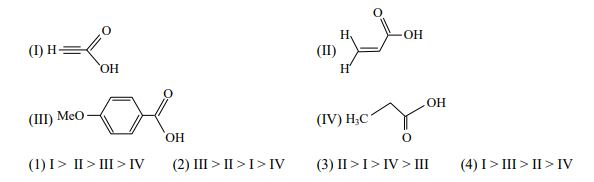Solution: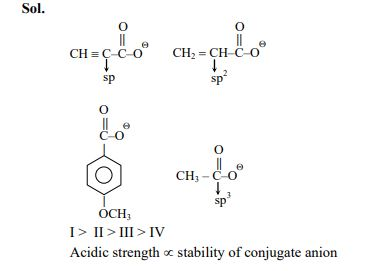3. Sodium stearate is a strong electrolyte. Which of the following plot is correct regarding its conductance: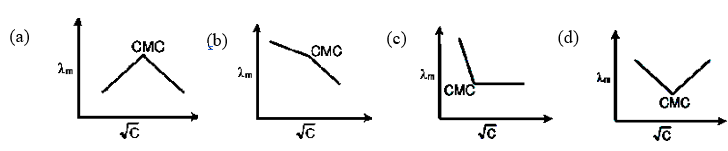Solution:

1. By definition, λmα (1/√C)

4. Which green coloured compound of chromium is formed in borax bead test?

1. a) Cr(BO2)3
2. b) Cr2O3
3. c) CrB
4. d) CrBO3

Solution:

1.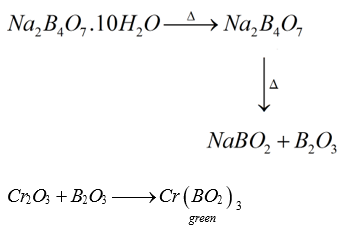5. Choose the reaction, for which the standard enthalpy of reaction is equal to the standard enthalpy of formation: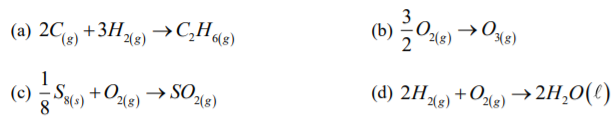Solution:

1. By definition,

Enthalpy of formation is defined as the Enthalpy change occurring when, a compound is formed from its constituent elements in standard state.

6. A Tin-chloride ‘P’ gives following reaction (unbalanced reaction)

P + Cl-

$$\rightarrow$$
X [Monoanion pyramidal geometry]

P + Me3N

$$\rightarrow$$
Y

P + CuCl2

$$\rightarrow$$
Z + CuCl

Then which of the following is/are correct.

1. a) Y contains co-ordinate bond
2. b) X is sp3 hybridised.
3. c) Oxidation state of Sn is X is +1.
4. d) X contain lone pair on central atom.

Solution:

1.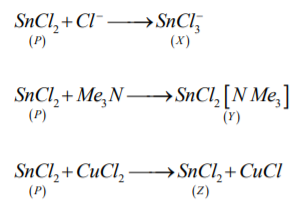7.

$$^{238}_{92}U \overset{x_1}{\rightarrow}^{234}_{90}Th \overset{x_2}{\rightarrow}^{234}_{91}Pa \overset{x_3}{\rightarrow}^{234}Z\overset{x_4}{\rightarrow}^{230}_{90}Th$$

x1, x2, x3, x4 are either particles or radiation. Then

1. a) x1 is deflected toward negatively charged plate.
2. b) x2 is β-particle.
4. d) z is isotope of 238U

Solution:

1. x1 -> α - decay

x2 -> β - decay

x3 -> β - decay

x4 -> α – decay

8. Fusion of MnO2 along with KOH and O2 forms X. Electrolytic oxidation of X yields Y. X undergoes disproportionation reaction in acidic medium to MnO2 and Y. The Manganese in X and Y is in the form W & Z respectively, then

1. a) W & Z are coloured
2. b) W is diamagnetic and Z is paramagnetic
3. c) Both W & Z are tetrahedral in shape
4. d) Both W & Z involve pπ-dπ bonding for π bond

Solution:

1.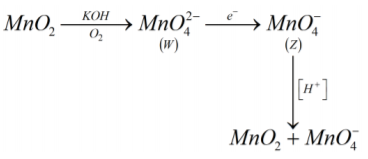9. Choose the corection option for the following reaction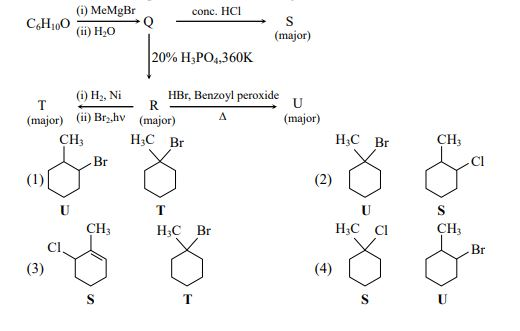Solution:

1.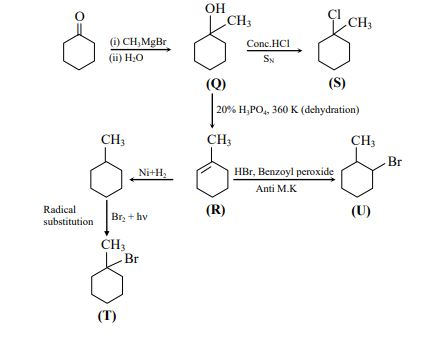10. Which of the following are true.

1. a) Monosachharides can not be hydrolysed to give polyhydroxy aldehydes and ketones.
2. b) Hydrolysis of sucrose gives dextrorotatory glucose and laevorotatory fructose
3. c) Oxidation of glucose with bromine water gives glutamic acid.
4. d) The two six membered hemiacetal form of D(+) glucose are anomers.

Solution:

1. Answer is: (a, b, d)

11. Identify the option where all four molecules possess permanent dipole moment at room temperature.

1. a) BF, O3, SF6, XeF6
2. b) BeCl2, CO2, BCl3, CHCl3
3. c) SO2, C6H5Cl, H2Se, BrF5
4. d) NO2, NH3, POCl3, CH3Cl

Solution:

1. For Option c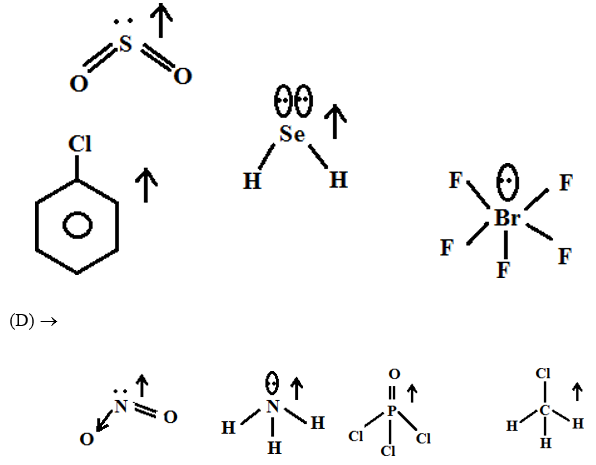12. Which of the following is/are correct regarding root mean square speed (Urms) & average translation K.E. (Eav) of molecule in a gas at equilibrium.

1. a) Eav is doubled when its temperature is increased 4 times
2. b) Urms is inversely proportional to the square root of its molecular mass
3. c) Eav at a given temperature doesn’t depend on its molecular mass
4. d) Urms is doubled when its temperature is increased 4 times

Solution:

1. Eav = (3/2)RT [independent of mass)

urms =

$$\sqrt{\frac{3RT}{M}}$$

13. XeF4 + O2F2

$$\rightarrow$$
product. The total number of lone pairs on the xenon containing product is: (l)

Solution:

1. 19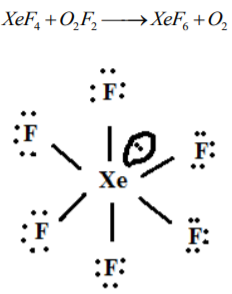Distorted octahedral shape.

14. For the following reaction, equilibrium constant Kc at 298 K is 1.6 × 1017.

$$Fe^{2+}_{(aq)} + S^{2-}_{(aq)} \rightleftharpoons FeS \ (s)$$

When equal volume of 0.06 M Fe+2 and 0.2 M S-2 solution are mixed, then equilibrium concentration of Fe+2 is found to be Y × 10-17 M. Y is:

Solution:

1. $$Fe^{2+} + S^{2-} \rightarrow FeS$$

 Ini 0.06 0.2 0 After mix 0.03 0.1 At 0 q/m x 0.07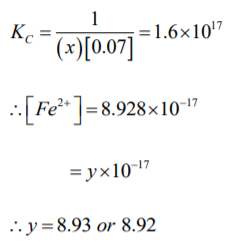15.Schemes 1 and 2 describe the conversion of P to Q and R to S, respectively. Scheme 3 describes the synthesis of T from Q and S. The total number of Br atoms in a molecule of T is _____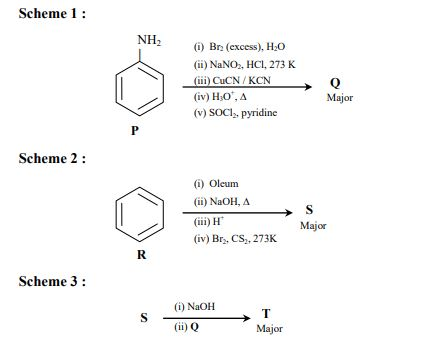Solution:

1.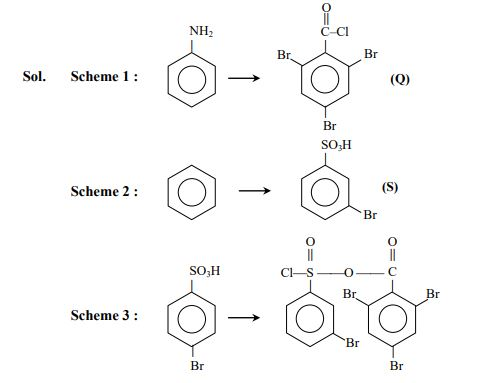Total Br atoms = 4

16. Which of the following compounds contain bond between same type of atoms.

N2O4, B3 N3H6, H2S2O3, N2O, H2S2O8, B2H6

Solution:

1.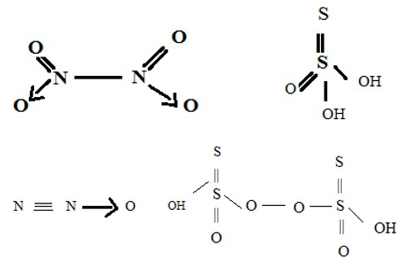17. Consider the kinetic data given in the following table for the reaction A + B + C → Product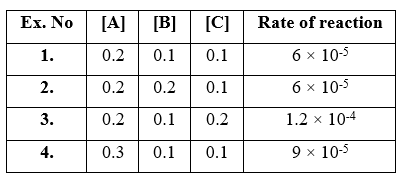When [A] = 0.15

[B] = 0.25

[C] = 0.15

Rate of reaction is Y × 10-5 m/s. Find Y.

Solution:

1. Let

$$r = K[A]^x[B]^y[C]^z$$

From (1), (2) -> y = 0

(1), (3) -> z = 1

(1), (4) -> x = 1

Therefore, rate law becomes

$$r = K[A]^1[B]^0[C]^1$$

From (2)

K = 3 x 10-3

When [A] = 0.15, [B] = 0.25, [C] = 0.15

r = 3 x 10-3 [0.15]1 [0.15]1

= 6.75 x 10-5 moll-1 s-1

So, y x 10-6

Hence, y = 6.75

18. On dissolving 0.5 g of non-volatile, non-ionic solute to 39 g of benzene, its vapour pressure decreases from 650 mm of Hg to 640 mm of Hg. The depression of freezing point of benzene (in K) upon addition of the solute is __________________.

[Given data: Molar mass & molar freezing point depression of benzene is 78 g mol-1 & 5.12 K Kg mol-1]

Solution:

1. $$\frac{P^0 - P_S}{P_S} = \frac{n_{solute}}{n_{solvant}}$$

$$\frac{650 - 640}{640} - \frac{1 \times 0.58 \times 78}{M \times 39}$$

Therefore, M = 64 g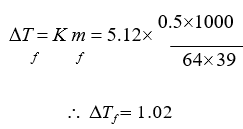### JEE Advanced 2019 Chemistry Paper 1 Solutions